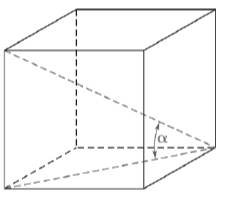Chapter 11.3, Problem 42EElementary Geometry For College St...

7th Edition
Alexander + 2 others
ISBN: 9781337614085

Solutions

Chapter
SectionElementary Geometry For College St...

7th Edition
Alexander + 2 others
ISBN: 9781337614085
Textbook Problem

In Exercises 39 to 45, angle measures should be given to the nearest degree; distance should be given to the nearest tenth of a unit.What is the measure of the angle between the diagonal of a cube and the diagonal of the face of the cube?To determine

To calculate:

The angle between the diagonal of a cube and the diagonal of the face of the cube.

Explanation

Given:

The provided figure is shown below:

Calculation:

Now, we have to find the angle between the diagonal of a cube and the diagonal of the face of the cube.

sinα=oppositehypotenuse.

Substitute hypotenuse=D,opposite=a

From the figure diagonal of the cube D=a3

Still sussing out bartleby?

Check out a sample textbook solution.

See a sample solution

The Solution to Your Study Problems

Bartleby provides explanations to thousands of textbook problems written by our experts, many with advanced degrees!

Get Started

Find more solutions based on key concepts